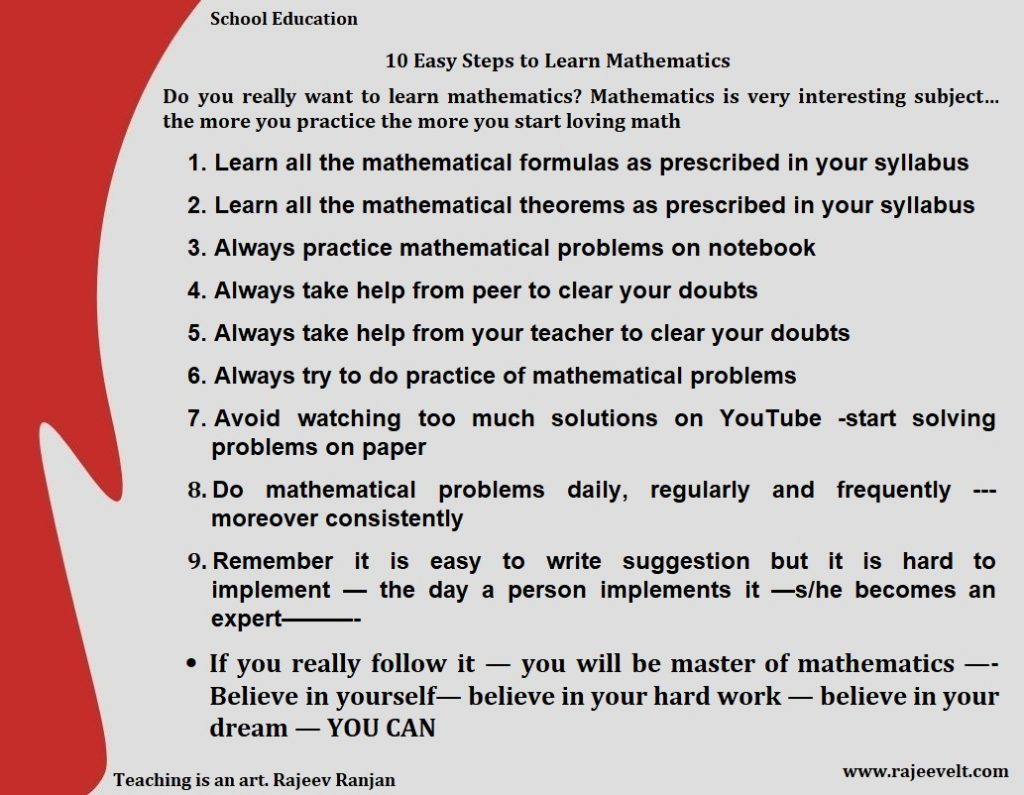Posted on

## 10 Easy Steps to Learn Mathematics- Students’ Problems and their Easy Solutions – Study TipsWhat are the steps to learn advance mathematics?

Hey Friend

Do you really want to learn mathematics? Mathematics is very interesting subject… the more you practice the more you start loving math————

1. Learn all the mathematical formulas as prescribed in your syllabus
2. Learn all the mathematical theorem as prescribed in your syllabus
3. Always practice mathematical problems on notebook
4. Always take help from peer to clear your doubts
5. Always take help from your teacher to clear your doubts
6. Always try to do practice of mathematical problems
7. Avoid watching too much solutions on Youtube -start solving problems on paper
8. Do mathematical problems daily, regularly and frequently —-moreover consistently
9. Remember it is easy to write suggestion but it is hard to implement — the day a person implement it —s/he becomes an expert———-
• If you really follow it — you will be master of mathematics —- Believe in yourself— believe in your hard work — believe in your dream — YOU CAN10 Easy Steps to Learn Mathematics- Students’ Problems and their Easy Solutions – Study Tips

https://www.quora.com/What-are-the-steps-to-learn-advance-mathematics/answer/Rajeev-Ranjan-2732

Posted on

## Math Study Tips and Tricks for Scoring Good Marks in Board Exam –15 Simple and Amazing Ideas to Learn MathematicsLow confidence in Math subject during the exam is common phenomena with board aspirant students. Students often commit silly mistakes in the final exam. Mathematics demands consistent practice and comprehensive conceptual knowledge to score good marks in the board examination. Peer assessment helps one another student to identify his own mistakes on the answer sheet which happened intentional or un-intentionally.

Generally board aspirant solve “Math’s Sample Paper” before the final board examination to have a command on Mathematics. In fact it helps students to develop habit of solving actual question paper comfortably and students get answers of exam related  following queries like (a) how to solve paper in within three hours (b) what are the common mistake occur during the examination (c) how to solve difficult question (d) which section should be start 1st in the examination (e) what should student do when s/he feels inability to solve a particular problem (f) what are other common mistakes which a student commits in the examination in Math (g) how to do time management (h) what are common ideas to overcome difficulties and score good marks in Math.

For finding the root cause of these above mentioned problems, we initiated sample paper practice programme in an artificially created exam center. We were stick with time only. There was no invigilator in the classroom to help students. Students have to solve paper in 3 hours. We asked our students to do peer assessment of solved sample paper for 45 minutes and instructed mathematics teacher for evaluating their students’ answer sheet. We asked each student to discuss his/her problems while s/he was solving Math’s’ sample paper.

We find some common problems with each and every student which we listed here. These are as following

 Problems Description/reason of problem Conceptual problem Solving mathematical problem in wrong way due to lack of comprehension Calculation problem Wrong calculation, jump over final answer Language problem Faced problems in mathematical tricky question Formulas problem Forget formula/written another formula Theorem problem/Essential situation for theorem Confused in theorem, do not write essential situations of theorem Construction problem Write mathematical construction in incorrectly Unit problem Wrong calculation, over calculation, leave calculation, jump on final calculation Diagram/Figure Habit of drawing wrong figure and diagram, putting wrong values Specific problem in probability, statics, Question asks to solve ‘mean’- student proved mean, medium, mode Confused in probability based question(what to do) Presentation on answer sheet Habit of leave question Incomplete/half done/half done then cross whole derivations/steps, over writing, Time Management Giving so much time on 1-3 marks questions, trying to solve a single question 2-3 times/focus on single question

Generally 20-30%, Mathematics questions are tricky and also require elaborate steps during solving these questions. In fact students commit many mistakes due to their wrong attitude towards the subject. Either we consider Math paper too easy or we create havoc on ourselves for its complexity. It is observed during peer assessment that students become excited when they find math’ question paper is easy which finally leads to silly mistakes like missing steps, simple calculation errors etc, or they become unnecessarily nervousness and fearful. Mathematician feels that students commits mistakes in mathematics due to  lack of Practice, lack of concentrationexam fear and pressure, mental tiredness, limited time and an effort to solve questions quickly.

How to Avoid Mistakes for Scoring Good Marks in Math

Magical Tips and Trick Only for You Because It is only You Who Have to Implement It

Relax and Be Confident– If you consider above written points, integrated in your mathematics learning process and indeed, you have studied reasonably; have been doing practice prescribed math syllabus consistently and continuously; you will shine like star in Mathematics. Last but not least cheer yourself and use your presence of mind, your logic and of course your common sense and your firm faith in God…………… If you are ———————————————————–! Mathematics is not more complex than your firm determination and your hard work. Mathematical complexity melts in the hand of hard worker. If you are!

Rajeev Ranjan